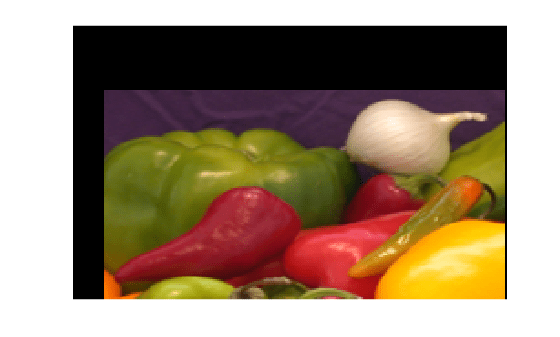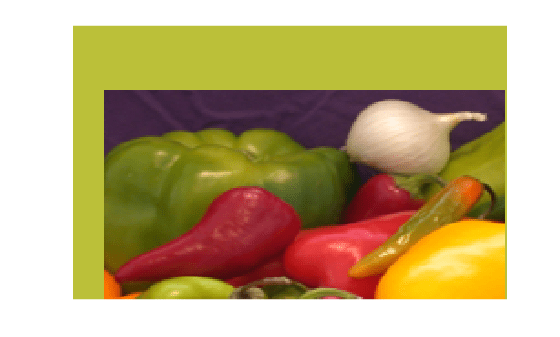# Specify Fill Values in Geometric Transformation Output

This example shows how to specify the fill values used by `imwarp` when it performs a geometric transformation. When you perform a transformation, there are often pixels in the output image that are not part of the original input image. These pixels must be assigned some value, called a fill value . By default, `imwarp` sets these pixels to zero and they display as black. Using the `FillValues` parameter, you can specify a different color. If the image being transformed is a grayscale image, specify a scalar value that specifies a shade of gray. If the image being transformed is an RGB image, you can use either a scalar value or a 1-by-3 vector. If you specify a scalar, `imwarp` uses that shade of gray for each plane of the RGB image. If you specify a 1-by-3 vector, `imwarp` interprets the values as an RGB color value.

Read image into workspace. This example uses a color image.

`rgb = imread('onion.png');`

Create the transformation matrix. This matrix defines a translation transformation.

```xform = [ 1 0 0 0 1 0 40 40 1 ];```

Create the geometric transformation object. This example creates an affine2d object.

`tform_translate = affine2d(xform)`
```tform_translate = affine2d with properties: T: [3x3 double] Dimensionality: 2 ```

Create a 2D referencing object. This object specifies aspects of the coordinate system of the output space so that the area needing fill values is visible. By default, `imwarp` sizes the output image to be just large enough to contain the entire transformed image but not the entire output coordinate space.

```Rout = imref2d(size(rgb)); Rout.XWorldLimits(2) = Rout.XWorldLimits(2)+40; Rout.YWorldLimits(2) = Rout.YWorldLimits(2)+40; Rout.ImageSize = Rout.ImageSize+[40 40];```

Perform the transformation with the `imwarp` function.

```cb_rgb = imwarp(rgb,tform_translate,'OutputView',Rout); figure, imshow(cb_rgb)```Now perform the transformation, this time specifying a fill value.

```cb_fill = imwarp(rgb,tform_translate,'FillValues',[187;192;57],... 'OutputView',Rout); figure, imshow(cb_fill)```